## Dice

A die (plural dice'') is a Solid with markings on each of its faces. The faces are usually all the same shape, making Platonic Solids and Archimedean Solid Duals the obvious choices. The die can be rolled'' by throwing it in the air and allowing it to come to rest on one of its faces. Dice are used in many games of chance as a way of picking Random Numbers on which to bet, and are used in board or role-playing games to determine the number of spaces to move, results of a conflict, etc. A Coin can be viewed as a degenerate 2-sided case of a die.

The most common type of die is a six-sided Cube with the numbers 1-6 placed on the faces. The value of the roll is indicated by the number of spots'' showing on the top. For the six-sided die, opposite faces are arranged to always sum to seven. This gives two possible Mirror Image arrangements in which the numbers 1, 2, and 3 may be arranged in a clockwise or counterclockwise order about a corner. Commercial dice may, in fact, have either orientation. The illustrations below show 6-sided dice with counterclockwise and clockwise arrangements, respectively.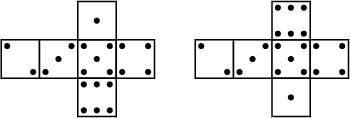The Cube has the nice property that there is an upward-pointing face opposite the bottom face from which the value of the roll'' can easily be read. This would not be true, for instance, for a Tetrahedral die, which would have to be picked up and turned over to reveal the number underneath (although it could be determined by noting which number 1-4 was not visible on one of the upper three faces). The arrangement of spots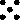corresponding to a roll of 5 on a six-sided die is called the Quincunx. There are also special names for certain rolls of two six-sided dice: two 1s are called Snake Eyes and two 6s are called Boxcars.

Shapes of dice other than the usual 6-sided Cube are commercially available from companies such as Dice & Games, Ltd.Diaconis and Keller (1989) show that there exist fair'' dice other than the usual Platonic Solids and duals of the Archimedean Solids, where a fair die is one for which its symmetry group acts transitively on its faces. However, they did not explicitly provide any examples.

The probability of obtaining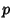points (a roll of) on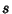-sided dice can be computed as follows. The number of ways in whichcan be obtained is the Coefficient of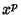in(1)

since each possible arrangement contributes one term.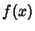can be written as a Multinomial Series(2)

so the desired number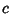is the Coefficient ofin(3)

Expanding,(4)

so in order to get the Coefficient of, include all terms with(5)is therefore(6)

But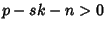only when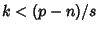, so the other terms do not contribute. Furthermore,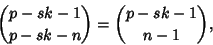(7)

so(8)

where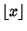is the Floor Function, and(9)

Consider now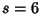. For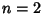six-sided dice,(10)

and(11)

The most common roll is therefore seen to be a 7, with probability, and the least common rolls are 2 and 12, both with probability 1/36.

For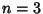six-sided dice,(12)

and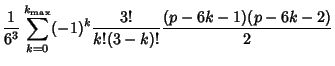(13)

For three six-sided dice, the most common rolls are 10 and 11, both with probability 1/8; and the least common rolls are 3 and 18, both with probability 1/216.

For four six-sided dice, the most common roll is 14, with probability 73/648; and the least common rolls are 4 and 24, both with probability 1/1296.

In general, the likeliest roll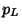for-sided dice is given by(14)

which can be written explicitly as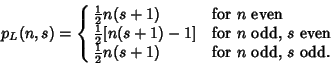(15)

For 6-sided dice, the likeliest rolls are given by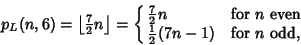(16)

or 7, 10, 14, 17, 21, 24, 28, 31, 35, ... for, 3, ... (Sloane's A030123) dice. The probabilities corresponding to the most likely rolls can be computed by plugging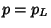into the general formula together with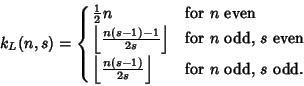(17)

Unfortunately,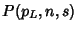does not have a simple closed-form expression in terms ofand. However, the probabilities of obtaining the likeliest roll totals can be found explicitly for a particular. For6-sided dice, the probabilities are 1/6, 1/8, 73/648, 65/648, 361/3888, 24017/279936, 7553/93312, ... for, 3, ....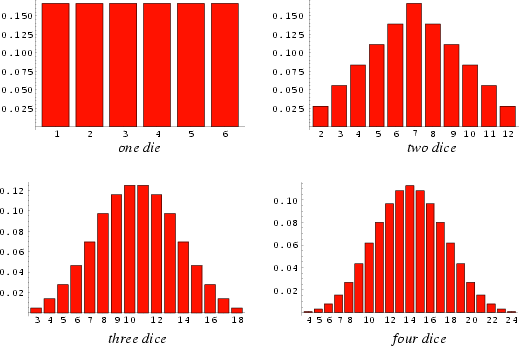The probabilities for obtaining a given total using6-sided dice are shown above for, 2, 3, and 4 dice. They can be seen to approach a Gaussian Distribution as the number of dice is increased.

See also Boxcars, Coin Tossing, Craps, de Mere's Problem, Efron's Dice, Poker, Quincunx, Sicherman Dice, Snake Eyes

References

Diaconis, P. and Keller, J. B. Fair Dice.'' Amer. Math. Monthly 96, 337-339, 1989.

Dice & Games, Ltd. Dice & Games Hobby Games Accessories.'' http://www.dice.co.uk/hob.htm.

Gardner, M. Dice.'' Ch. 18 in Mathematical Magic Show: More Puzzles, Games, Diversions, Illusions and Other Mathematical Sleight-of-Mind from Scientific American. New York: Vintage, pp. 251-262, 1978.

Robertson, L. C.; Shortt, R. M.; Landry, S. G. Dice with Fair Sums.'' Amer. Math. Monthly 95, 316-328, 1988.

Sloane, N. J. A. Sequence A030123 in The On-Line Version of the Encyclopedia of Integer Sequences.'' http://www.research.att.com/~njas/sequences/eisonline.html.